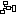# Code Smells

Code Smells / Bloaters / Data Clump# Data Clump

Data clumps are sets of related primitives (eg. `1`, `3.14`, `"Hello"`, `False`) that always appear together. Data Clumps can be avoided by encapsulating them together in a class.

``````from math import sqrt

# Code smell
x_coordinate = 4
y_coordinate = -7
z_coordinate = 43

distance = sqrt(x_coordinate**2 + y_coordinate**2 + z_coordinate**2)  # Pythagorean theorem
print(distance)

# Improved
class Coordinates:
def __init__(self, x_coordinate, y_coordinate, z_coordinate):
self.x_coordinate = x_coordinate
self.y_coordinate = y_coordinate
self.z_coordinate = z_coordinate

def distance(self):
return sqrt(self.x_coordinate**2 + self.y_coordinate**2 + self.z_coordinate**2)  # Pythagorean theorem

my_coordinates = Coordinates(4, -7, 43)
print(my_coordinates.distance())
``````

The problem with Data Clumps, is that it is not easy to centrally control their behaviour. In the example above, if we wanted to restrict `y_coordinate` to positive numbers only, we may need to edit the code in many places, leading to Shotgun Surgery.

Data Clumps are also often Primitive Obsessions.